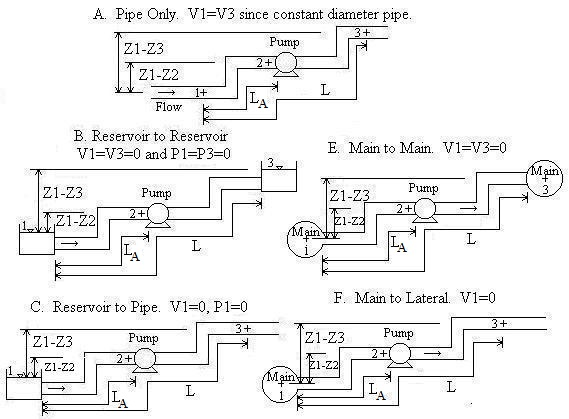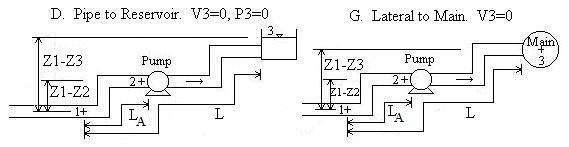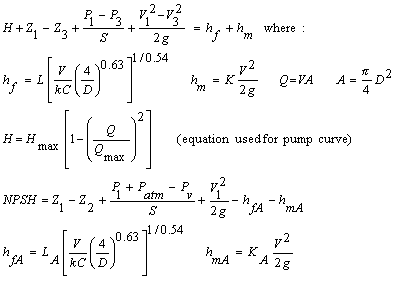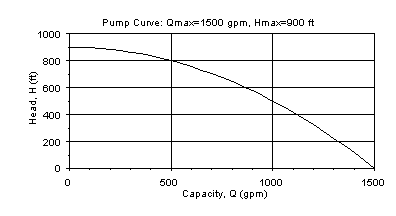Circular Pressurized Water Pipe with Pump Curve (Hazen-Williams)

Parabolic-shaped pump curve. Water at temperature typical of city water supply systems (40 to 75 oF; 4 to 25 oC).

Register to enable "Calculate" button.

 Inputs/Outputs: Flow Rate, Q (gpm): Velocity: Vpipe (ft/s): Select Calculation and Scenario: Pipe Inside Diameter, D (inch): Solve for V, Q Q known. Solve for Z1-Z3 Q known. Solve for P1-P3 Q known. Solve for Diameter Q known. Solve for Pipe Length Q known. Solve for C Q known. Solve for K V known. Solve for Z1-Z3 V known. Solve for P1-P3 V known. Solve for Diameter V known. Solve for Pipe Length V known. Solve for C V known. Solve for K Total Length, L (ft): A - Pipe Only B - Reservoir to Reservoir C - Reservoir to Pipe D - Pipe to Reservoir E - Main to Main F - Main to Lateral G - Lateral to Main Hazen-Williams Coefficient, C: Select Units: Minor Loss Coeff for Entire Pipe, K: Flow in m3/s Flow in ft3/s (cfs) Flow in U.S.gal/min (gpm) Flow in U.S.gal/day (gpd) Elevation Difference, Z1-Z3 (ft): Velocity in m/s Velocity in ft/s Pressure Diff., P1-P3 (psi): Diameter in m Diameter in cm Diameter in ft Diameter in inch Inputs for pump and NPSH: L, Z, H, DH, hf, hm, NPSH in m L, Z, H, DH, hf, hm, NPSH in ft Pump's max Q, Qmax (gpm): P1-P3, P1 (gage) in N/m2 (Pa) P1-P3, P1 (gage) in bar P1-P3, P1 (gage) in m of water P1-P3, P1 (gage) in lb/ft2 (psf) P1-P3, P1 (gage) in lb/in2 (psi) P1-P3, P1 (gage) in ft of water Pump's max Head, Hmax (ft): Atm Pressure in Pa Atm Pressure in bar Atm Pressure in mm Hg (0C) Atm Pressure in atm Atm Pressure in psi Atm Pressure in inch Hg (60F) Length of Pipe A, LA (ft): Power in N-m/s (Watt) Power in ft-lb/s Power in horsepower (hp) Minor Loss Coeff for Pipe A, KA: Elevation Difference, Z1-Z2 (ft): http://www.LMNOeng.com Pressure at 1, P1 (psig): © 2015 LMNO Engineering, Atm Pressure, Patm (psi): Research, and Software, Ltd. Always Computed: Total Dynamic Head, H (ft): Net Pos Suction Head, NPSHA (ft): Pump Power (hp): Driving Head, DH (ft): Major (friction) Loss, hf (ft): Minor Loss, hm (ft):

Software computes flow rate (i.e. discharge, capacity), velocity, pipe diameter, length, elevation difference, pressure difference, major losses (Hazen Williams), minor losses, total dynamic head, net positive suction head.

Units: cfs=cubic foot per second, cm=centimeter, ft=foot, gal=U.S. gallon, gpd=U.S. gallon per day, gpm=U.S. gallon per mimute, hp=horsepower, lb=pound, m=meter, min=minute, N=Newton, Pa=Pascal, psi=lb/inch2, s=second

Introduction
The Hazen-Williams equation for major (friction) losses is commonly used by engineers for designing and analyzing piping systems carrying water at typical temperatures of municipal water supplies (40 to 75 oF; 4 to 25 oC). A pump curve is incorporated into the calculation to simulate flows containing centrifugal pumps or other pumps that have a pump curve. To keep the calculation's input relatively simple, we only require you to enter two points on the pump curve - flow at zero head and head at zero flow. A parabolic curve is then formed between the two points as shown in Equations below. The calculation also asks for information specifically about the pipe on the suction side of the pump. This information is used to compute the net positive suction head available (NPSHA). For a pump to properly function, the NPSHA must be greater than the NPSH required by the pump (obtained from the pump manufacturer). If your system does not require a pump or uses a pump that does not have a parabolically shaped pump curve, then our other Hazen-Williams design calculation may be more helpful.

Piping Scenarios
Pipe A is the pipe upstream from the pump (i.e. the suction side pipe).
Convention for Z1-Z2 and Z1-Z3: If location 1 is above location 2, then Z1-Z2 should be entered as positive. If location 2 is above location 1, then Z1-Z2 should be entered as negative. Likewise for Z1-Z3.Equations and Methodology                                     Back to Calculations
The calculation on this page uses the steady state energy equation. Minor losses (due to valves, pipe bends, etc.) and major losses (due to pipe friction) are included. The Hazen-Williams equation for friction losses is used. The equations are standard equations which can be found in most fluid mechanics textbooks (see References). A pump curve is included in the calculation. Determination of the pump curve requires that the user enter the two extreme points on the curve - head when capacity is zero, and capacity when head is zero. Then, a parabola with a negative curvature is fit through the two points. This parabola is used since it is a good approximation of a typical pump curve and does not require users to enter a multitude of data points. And, oftentimes, pump catalogs only give the two extreme points on the curve rather than a graph showing the complete curve.Pump Curve
To provide an example of a pump curve developed using the equation H=Hmax[1-(Q/Qmax)2], let Qmax=1500 gpm (when head is zero) and Hmax=900 ft (when Q is zero). The pump curve used in the calculation will look like:All of the calculations on this page have analytic (closed form) solutions except for "Solve for V, Q", "Q known. Solve for Diameter", and "V known. Solve for Diameter". These three calculations required a numerical solution. Our solution utilizes a modified implementation of Newton's method that finds roots of the equations with the result accurate to 8 significant digits. All of the calculations utilize double precision, but results are shown to eight significant figures. "V known. Solve for Diameter" may find two diameters which give the same velocity - if this is the case, both diameters are shown.

Variables   Units:  L=length, P=pressure, T=time                    Back to Calculations
A = Pipe area [L2].
C = Hazen-Williams coefficient. See table below.
D = Pipe inside diameter [L].
DH = Driving Head [L] = left side of the first equation above. Also equals the right side of the equation which is the sum of major and minor losses.
g = Acceleration due to gravity = 32.174 ft/s2 = 9.8066 m/s2
hf = Major losses for entire pipe [L].
hfA = Major losses for pipe upstream of pump (pipe A) only [L].
hm = Minor losses for entire pipe [L].
hmA = Minor losses for pipe upstream of pump (pipe A) only [L].
Hmax = Maximum head that pump can provide [L]. It is the head when Q=0.
k = Unit conversion factor = 1.318 for English units = 0.85 for Metric units.
K = Sum of minor loss coefficients for entire pipe. See table below for values.
KA = Sum of minor loss coefficients for pipe upstream of pump (pipe A).
L = Total pipe length [L].
LA = Length of pipe upstream of pump (pipe A) [L].
NPSHA = Net positive suction head available [L].
Patm = Atmospheric (or barometric) pressure [P]. Standard atmospheric pressure = 14.7 psi = 29.92 inch Hg = 760 mm Hg = 1 atm = 101,325 Pa = 1.01 bar. Note that your local atmospheric pressure is different from standard atmospheric pressure.
Pv = Vapor pressure of fluid [P]. Expressed as an absolute pressure. This value is built-in to the program as 2000 N/m2 (absolute) for water at 15oC.
P1 = Gage pressure at location 1 of the system [P]. Location 1 could be the surface of a reservoir open to the atmosphere (thus P1=0), or the pressure in a supply main (same as a tank under pressure), or location 1 could simply be a location in a pipe upstream of the pump.
P1-P3 = Pressure difference between locations 1 and 3 [P].
Q = Flowrate [L3/T]. Also known as discharge or capacity.
Qmax = Maximum flowrate on pump curve [L3/T]. Corresponds to point on pump curve where head is zero.
S = Specific Weight of Water (i.e. weight density; weight per unit volume)
= 62.4 lb/ft3 for English units = 9800 N/m3 for Metric units
V1 = Velocity of fluid at location 1. This is determined when you select a scenario. If location 1 is a reservoir or main (Scenarios B, C, E, and F), then V1 is automatically set to 0 because the velocity head of the fluid in the reservoir or main (or pressure tank) is much smaller than in the attached pipeline. This is a standard assumption in fluid mechanics. However, if location 1 is inside the suction side pipeline, then V1 is automatically computed as Q/A.
V3 = Velocity of fluid at location 3. This is determined when you select a scenario. If location 3 is a reservoir or main (Scenarios B, D, E, and G), then V3 is automatically set to 0 because the velocity head of the fluid in the reservoir or main (or pressure tank) is much smaller than in the attached pipeline. This is a standard assumption in fluid mechanics. However, if location 3 is inside your discharge side pipeline, then V3 is automatically computed as Q/A.
Z1-Z2 = Elevation of location 1 minus elevation of pump [L]. If the pump is above location 1, then enter this value as negative.
Z1-Z3 = Elevation of location 1 minus elevation of location 3 [L].

Common Questions                                                       Back to Calculations
What is net positive suction head? It is the sum of the heads that push fluid into the pump less the suction side losses. Most pumps have a minimum requirement for NPSH, called NPSHR. If the NPSH available by the piping system (NPSHA) is lower than NPSHR, then the pump will not function properly and may overheat.
What is Driving Head? DH is the sum of heads supplied by the pump, elevation, pressure, and velocity differences between the inlet and outlet system boundaries. DH is equivalent to the sum of minor and major losses.
How is Total dynamic head different than Driving head? Total dynamic head, H, is the head that the pump must provide to overcome major losses, minor losses, and elevation, pressure, and velocity head differences between outlet and inlet. H may be more or less than DH depending on whether the elevation, pressure, and/or velocity head differences are beneficial or must be overcome.
Your program is great! What are its limitations? Pipes must all have the same diameter. The fluid must be water. Our approximation for the pump curve may not be close enough to your actual pump curve to give sufficiently accurate results.
Do you have more common questions and answers somewhere else on your website? Yes, see our Hazen-Williams calculation without pump curves.
Where can I find additional information? References

Table of Hazen-Williams Coefficients (C is unit-less)       Back to Calculations
Compiled from References

 Material C Material C Asbestos Cement 140 Copper 130-140 Brass 130-140 Galvanized iron 120 Brick sewer 100 Glass 140 Cast-Iron: Lead 130-140 New, unlined 130 Plastic 140-150 10 yr. old 107-113 Steel: 20 yr. old 89-100 Coal-tar enamel lined 145-150 30 yr. old 75-90 New unlined 140-150 40 yr. old 64-83 Riveted 110 Concrete/Concrete-lined: Steel forms 140 Tin 130 Wooden forms 120 Vitrif. clay (good condition) 110-140 Centrifugally spun 135 Wood stave (avg. condition) 120

Table of Minor Loss Coefficients (K is unit-less)       Back to Calculations
Compiled from References

 Fitting K Fitting K Valves: Elbows: Globe, fully open 10 Regular 90o, flanged 0.3 Angle, fully open 2 Regular 90o, threaded 1.5 Gate, fully open 0.15 Long radius 90o, flanged 0.2 Gate 1/4 closed 0.26 Long radius 90o, threaded 0.7 Gate, 1/2 closed 2.1 Long radius 45o, threaded 0.2 Gate, 3/4 closed 17 Regular 45o, threaded 0.4 Swing check, forward flow 2 Swing check, backward flow infinity Tees: Line flow, flanged 0.2 180o return bends: Line flow, threaded 0.9 Flanged 0.2 Branch flow, flanged 1.0 Threaded 1.5 Branch flow, threaded 2.0 Pipe Entrance (Reservoir to Pipe): Pipe Exit (Pipe to Reservoir) Square Connection 0.5 Square Connection 1.0 Rounded Connection 0.2 Rounded Connection 1.0 Re-entrant (pipe juts into tank) 1.0 Re-entrant (pipe juts into tank) 1.0

Error Messages                         Back to Calculations
Some or all values may be computed even with error messages, even though physically the situation cannot occur.
"An input is < 0." The following values must be entered as ≥ 0: K and KA. One or more of them was entered as <0.
"An input is ≤ 0." The following values must be entered as positive: Q, V, D, L, C, Qmax, Hmax, LA. One or more of them was entered as ≤0.
"KA must be ≤ K." Minor loss coefficient for pipe A cannot exceed the minor loss coefficient for the entire pipe system.
"LA must be ≤ L". The length of pipe A cannot exceed the length of the entire pipe.
"P1+Patm must be >0." The sum of P1+Patm gives P1 in absolute pressure. It is physically impossible to have an absolute pressure ≤ 0 since that implies a complete vacuum at location 1.
"Need P1-(P1-P3)+Patm >0." The absolute pressure at location 3 is less than 0 absolute. It is physically impossible to have a complete vacuum.
"Q must be ≤ Qmax." System flowrate cannot be entered as greater than the maximum flow rate that the pump can deliver.
"Tanks open so P1-P3=0 for B." This message occurs if Scenario B (reservoir to reservoir) is selected and Solve for P1-P3 is selected. Reservoirs are defined to be open to the atmosphere, so they have a pressure difference of zero by default. If you have tanks that are under pressure, select Scenario E (main to main) instead.
"Pump not needed. H will be ≤0." The system characteristics that were entered result in a negative total dynamic head which means that a pump is not necessary to deliver the flow. There are enough elevation, pressure, and/or velocity head differences to overcome the major and minor losses without the need of a pump. For this situation, it would be better to run our Hazen-Williams calculation that doesn't incorporate a pump curve.
"Infeasible Input. DH will be ≤0." Driving head (the left hand side of the first equation shown above in Equations) must be positive in order for fluid to flow. The system and pump characteristics entered result in DH being ≤ 0.
"Infeasible Input. (DH-hm)≤0." The difference (DH-hm) is ≤ 0 implying that major losses will also be ≤0 which is impossible for a flowing fluid.
"Infeasible Input (DH-hf )<0." The difference (DH-hf ) is < 0 implying that minor losses will also be <0, which is impossible.
"Infeasible input." Driving head and/or major losses are ≤0; or minor losses are < 0.

LMNO Engineering, Research, and Software, Ltd.
7860 Angel Ridge Rd.   Athens, Ohio  45701  USA   Phone: (740) 592-1890
LMNO@LMNOeng.com    http://www.LMNOeng.com

To:

Other single pipe calculators:

Darcy-Weisbach with pump curve

Hazen-Williams without pump curve

Darcy-Weisbach without pump curve

Multiple pipes:

Bypass Loop

Pipe Network

Videos of experiment and analysis of friction loss

Register